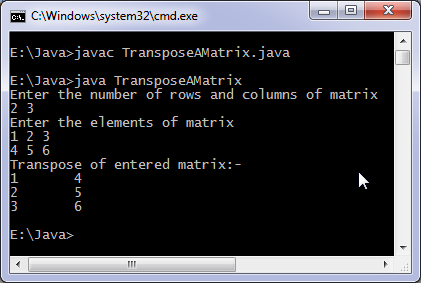# Java program to transpose matrix

Levels of difficulty: / perform operation:

This java program find transpose of a matrix of any order.

## This java program

```import java.util.Scanner;
class TransposeAMatrix {
public static void main(String args[]) {
int m, n, c, d;
Scanner in = new Scanner(System.in);
System.out.println("Enter the number of rows and columns of matrix");
m = in.nextInt();
n = in.nextInt();
int matrix[][] = new int[m][n];
System.out.println("Enter the elements of matrix");
for ( c = 0 ; c < m ; c++ )
for ( d = 0 ; d < n ; d++ )
matrix[c][d] = in.nextInt();
int transpose[][] = new int[n][m];
for ( c = 0 ; c < m ; c++ ) {
for ( d = 0 ; d < n ; d++ )
transpose[d][c] = matrix[c][d];
}
System.out.println("Transpose of entered matrix:-");
for ( c = 0 ; c < n ; c++ ) {
for ( d = 0 ; d < m ; d++ )
System.out.print(transpose[c][d]+"\t");
System.out.print("\n");
}
}
}
```

### OutputThis code can be used to check if a matrix symmetric or not, just compare the matrix with it’s transpose if they are same then it’s symmetric otherwise non symmetric, also it’s useful for calculating orthogonality of a matrix.